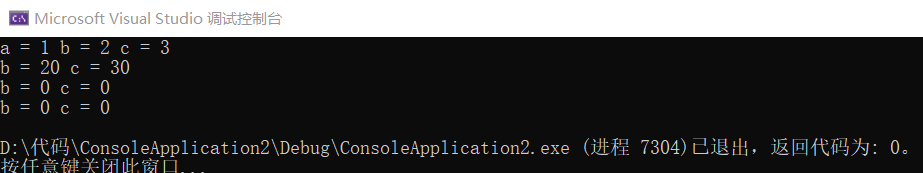# c++第四次作业

1.单继承

class Rectangle : public Point
{
public:
Rectangle();
~Rectangle();
};

2.多继承

class Derived : public Base1, private Base2
{
public:
Derived();
~Derived();
};


public（公有继承） 基类中的公有成员和保护成员都以公有成员身份出现在派生类中，而基类中的私有成员在派生类中不可直接访问。

protected（保护继承） 基类中的公有成员和保护成员都以保护成员身份出现在派生类中，而基类中的私有成员在派生类中不可直接访问。

private（私有继承）基类中的公有成员和保护成员都以私有成员身份出现在派生类中，而基类中的私有成员在派生类中不可直接访问。

#include <iostream>
using namespace std;

class A
{
private://私有成员
int a;
protected://保护成员
int b;
public://公有成员
int c;
A()//构造函数
{
a = 0;
b = 0;
c = 0;
}
void setA(int a, int b, int c)
{
this->a = a;
this->b = b;
this->c = c;
}
void print()
{
cout << "a = " << a << " b = " << b << " c = " << c << endl;
}
};

class B : public A//公有继承
{
public:
void print()
{
//cout<<"a = "<<a; //基类的私有成员不可直接访问
cout <<"b = " << b << " c = " << c << endl;
}
};

class C : protected A//保护继承
{
public:
void print()
{
//cout<<"a = "<<a; //基类的私有成员不可直接访问
cout << "b = " << b << " c = " << c << endl;
}
};

class D : private A//私有继承
{
public:
void print()
{
//cout<<"a = "<<a; //基类的私有成员不可直接访问
cout << "b = " << b << " c = " << c << endl;
}
};

int main()
{
A aa;
B bb;
C cc;
D dd;

aa.c = 100;
bb.c = 100;
//cc.c = 100; //在类的外部，保护继承将基类所有成员视为私有成员，不可访问
//dd.c = 100; //私有继承，基类中所有成员视为私有成员，不可访问

aa.setA(1, 2, 3);
bb.setA(10, 20, 30);
//cc.set(40, 50, 60); //在类的外部，保护继承将基类所有成员视为私有成员，不可访问
//dd.set(70, 80, 90); //私有继承，基类中所有成员视为私有成员，不可访问

aa.print();
bb.print();
cc.print();
dd.print();

return 0;
}1.吸收基类成员

2.改造基类成员

3.添加新的成员

posted @ 2019-10-13 18:20  zlx111  阅读(118)  评论(0编辑  收藏  举报